# Making Music with the Accelerometer

miniBloq
Easy

## Introduction

Although we have used different sensors like the ultrasonic distance ranger, the light sensors, or the line following sensors, there are more Sparki sensors to discover. One of them is the 3D accelerometer. The accelerometer can be used for a lot of things, but in this lesson, we will start to learn about it with a simple goal in mind: make music by moving Sparki with our hands.

## How It Works

If you are not familiar with Sparki’s accelerometer, please first read this description. The accelerometer commands provided in Sparki’s software will let us get to the acceleration data in the 3 spatial axes (X, Y and Z):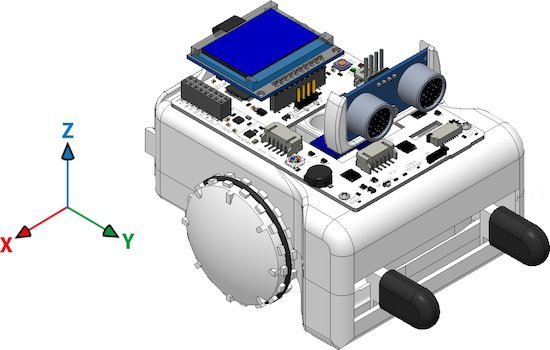These are the basic blocks to read the accelerations: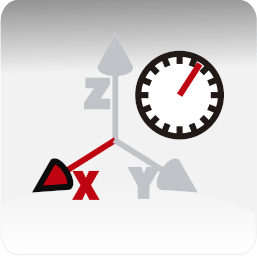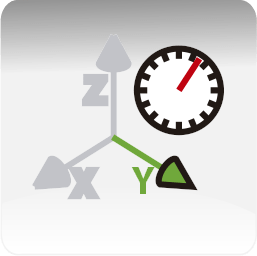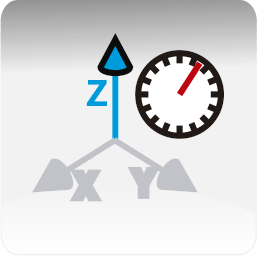For this activity, we will read the X and Y values and then we will generate musical notes with them. A musical note will be defined by two parameters: its frequency and its duration. So let’s first make different notes, which means modifying the frequency sent to Sparki’s buzzer but with a fixed duration. The accelerometer returns the acceleration values in m/s2. If you don’t understand this unit, don’t worry! You just need to know that the Earth’s gravity acceleration is approximately -9.8 m/s2. The minus sign indicates that the value is towards the Earth’s center. If you put your Sparki over a table as horizontal as possible, the X and Y will be near 0.0 (maybe with some noisy small variations), while the Z value will be around -9.8. But if you take the Sparki with your hands, and tilt it around its X axis (thus producing a variation in the Y and Z readings), the resulting values should similar to these (Note: we are working on a new version of the software that will let you see all these values on the screen, but by now, you can test this with SparkiDuino, as shown in this link):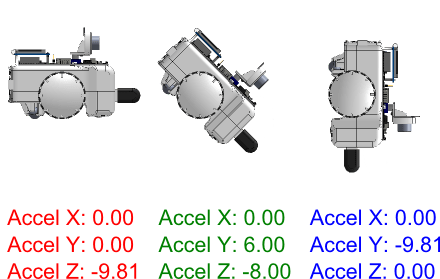So, let’s use the Y value to make the notes! The idea is pretty simple:
• Read the Y acceleration value from the accelerometer.
• Make that value to always be positive (since frequencies can not be negative!) using the abs function.
• Multiply that value for a number.  The number should be big enough to convert our Y reading in a frequency corresponding to an audible sound that can be sent to the buzzer. For example: a frequency of 440 Hz is the A note. So, let’s start multiplying the reading by 100. Thus if Sparki is fully vertical (in the Y axis), the frequency will be near 1000 Hz (or 1 KHz), since 9.8 x 100 = 980.
• Wait for some milliseconds (note’s duration) using the block belonging to the delay function.
Here is the code (we are just modifying the previous example, since viewing the accelerometer values is always useful):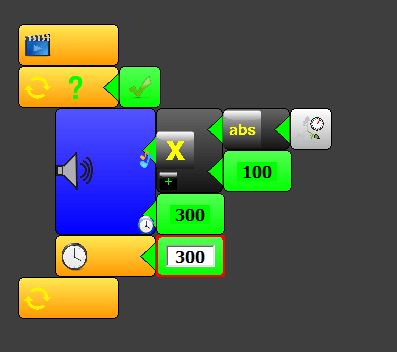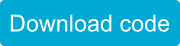You can experiment with that 100 value, increasing or decreasing it to create higher and lower notes. Finally, the “300” value (expressed in milliseconds) for both the duration in the speaker block and the value for the delay block, defines the duration of the note. So, to complete our musical instrument, we can change this line in a way so that the note duration is defined by the X value from the accelerometer instead of being a fixed number. Let’s try the following code: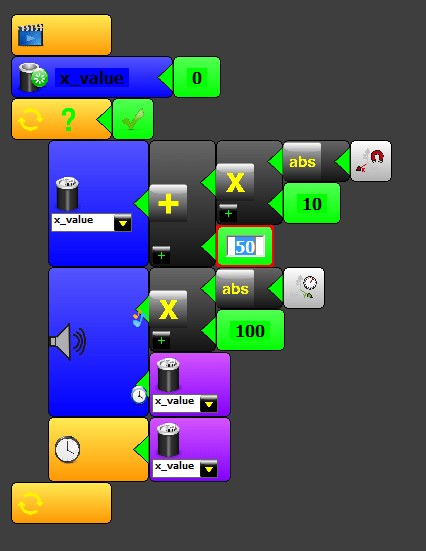Of course, you can now play with the numbers in that line too!